# General Room Demolition (square feet) - Used in Project

The general room demo (square feet) item assembly is based on a production rate of 25 square feet per hour. The Estimate Rocket smart line items output 3 sets of information when an item is added to a project:

1. Price and detailed work description for your customer to see
2. Estimated profit margin calculation
3. Time and materials calculations for work orders

Click here to see how to modify the General Room Demo (square feet) for specific use.

In this sample project a single room is going have demolition work done

Line Item is Added Directly to the Project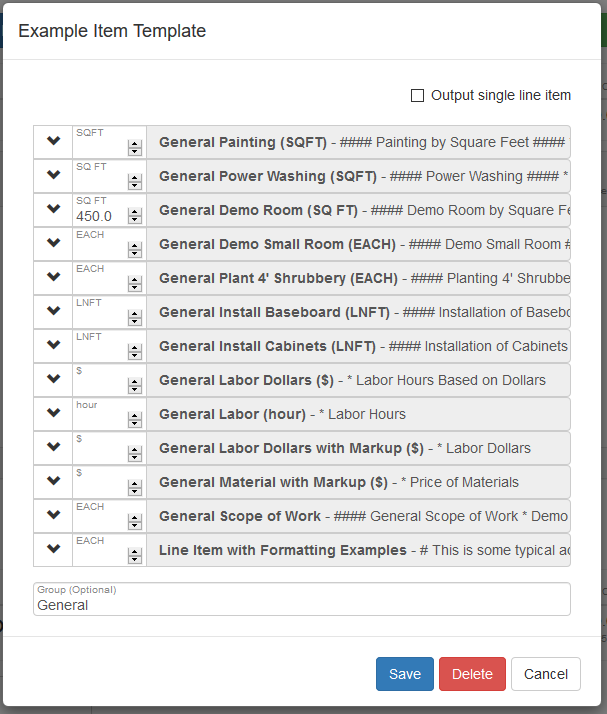• Room measurement = 450 square feet
• Production rate of demo = 25 square feet per hour
• Calculation 1 = 450 square feet / 25 square feet per hour = 18 hours needed
• Calculation 2 = 18 hours x \$50 per hour = \$900 total dollars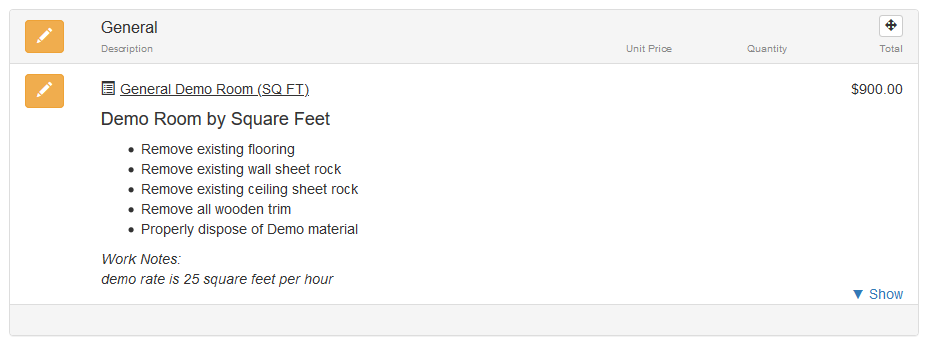The square footage room demo is priced at \$900 and the customer will see that price on their estimate along with a detailed description of the work to be done.

A Preview of the Estimate shows the customer the price and detailed description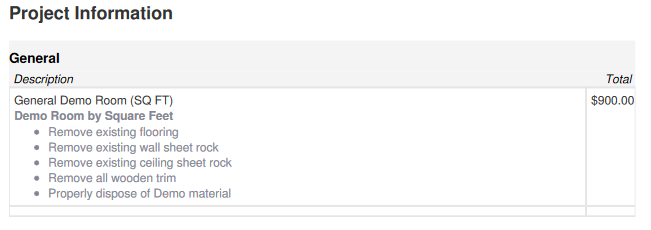Estimated Profit Margin

The single room demo also calculates an estimated profit margin for this project as seen in the project detail screen.In this case the only component involved in the assembly is a labor component which is priced to the customer at \$50 per hour but costs \$25 per hour. The profit margin for the room demo is 50% based on the default item setup.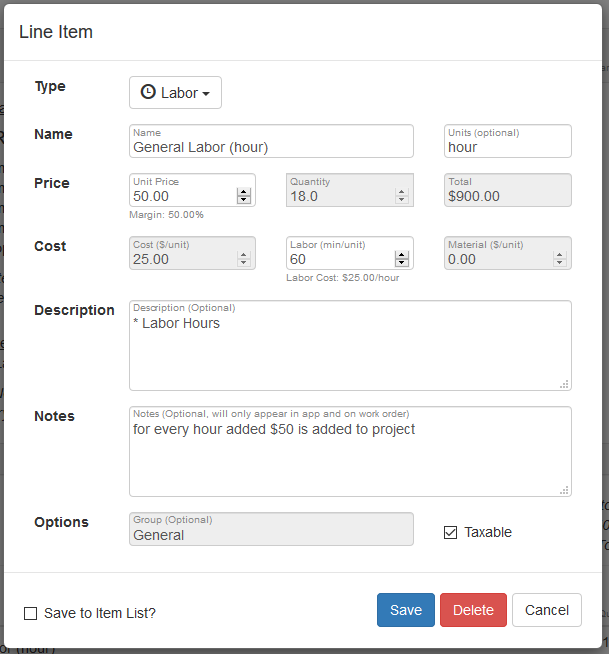The labor and materials are also calculated for in the work order. The labor and materials section is not displayed on the customer estimate but is displayed in the work order.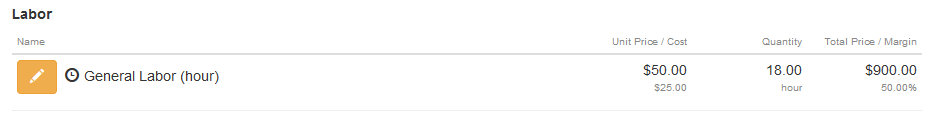In this case there are only labor hours included so only total hours are calculated.

Click here to see how to modify the General Room Demo (square feet) for specific use.